M7-S12: Relativistic Momentum and Energy-Mass Equivalence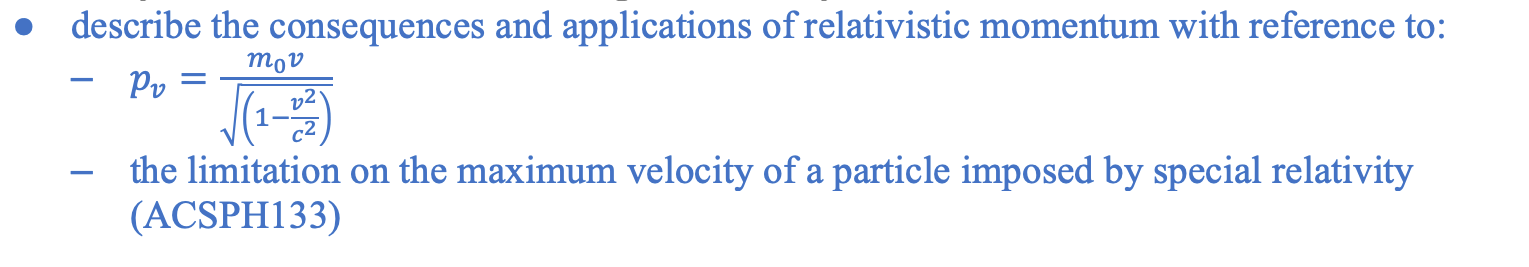Mass Dilation

To a stationary observer, the mass of a particle travelling at relativistic velocity becomes heavier (dilated).

Implications of mass dilation

• The main implication of mass dilation is that more energy is required to accelerate a body when its mass becomes so large at relativistic velocities.
• The energy required to accelerate a body to the speed of light is infinitely large, thus deeming the process impossible. This implication is demonstrated in particle accelerators whereby theoretical calculations indicate that to accelerate an electron to the speed of light would require more energy than what is available in this universe.
• Einstein also stated that the speed of light is the absolute limit in the universe in which it cannot be reached and exceeded. As time becomes slower at relativistic speeds, it would theoretically come to a halt at the speed of light. When time stops, the concept of speed (distance over time) becomes incomprehensible.

Relativistic Momentum

As the velocity of a body approaches the speed of light, its momentum increases according to classical momentum equation p = mv. However, classical momentum theory becomes inconsistent with experimental results when a body is travelling at a relativistic velocity. This is because an object’s mass increases along with its velocity.

Since Einstein’s special relativity theory only applies to inertial frames of reference, relativistic momentum must also obey the law of conservation of momentum (a classical physics law that applies in inertial reference frames).

Classical theory of momentum: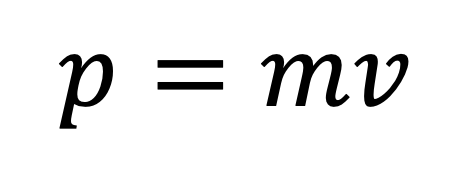Hence: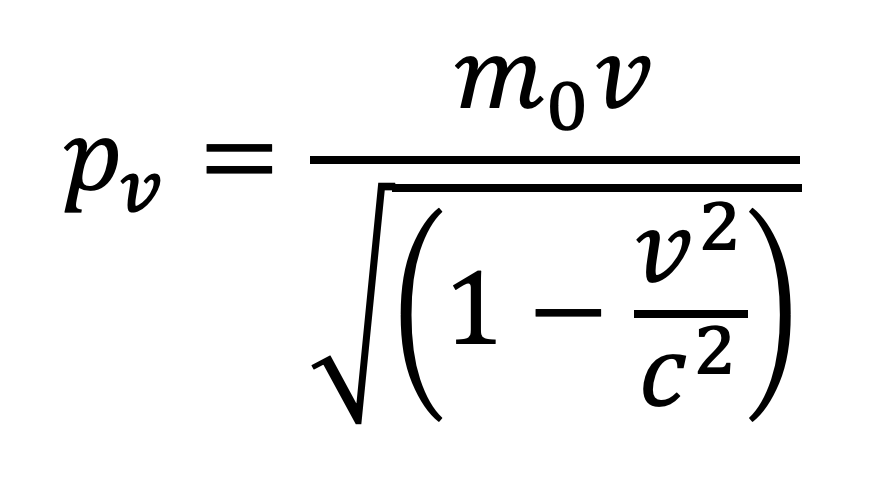• where pv is the relativistic momentum of a particle or body measured and observed from a moving frame of reference (or stationary observer’s perspective).
• m0 is the mass of the body measured by an observer in the rest frame. Rest frame is defined as a reference frame in which the observer is moving at the same velocity as the observed body.
• v is the relative velocity of the body as observed by a stationary observer.
• Relativistic momentum is measured using a body’s rest mass (m0) so the increase in momentum is only due to the increase in the body’s velocity.• Speed of light is the absolute limit of any body’s velocity. No objects in the universe can exceed this limit. While this imposes a limit on classical momentum (p = mv), relativistic momentum, in contrast, approaches to infinity as the velocity of a body approaches the speed of light.

Why is rest mass used to calculate relativistic momentum?

Relativistic momentum is measured using rest mass because mass of a moving body cannot be determined without analysing collision or any interactions involving momentum. This means relativistic mass cannot be determined without first finding a body’s momentum.

However, by using the mathematical relationship between rest mass and relativistic mass, we can indirectly determine the latter.

Relativistic versus Classical Momentum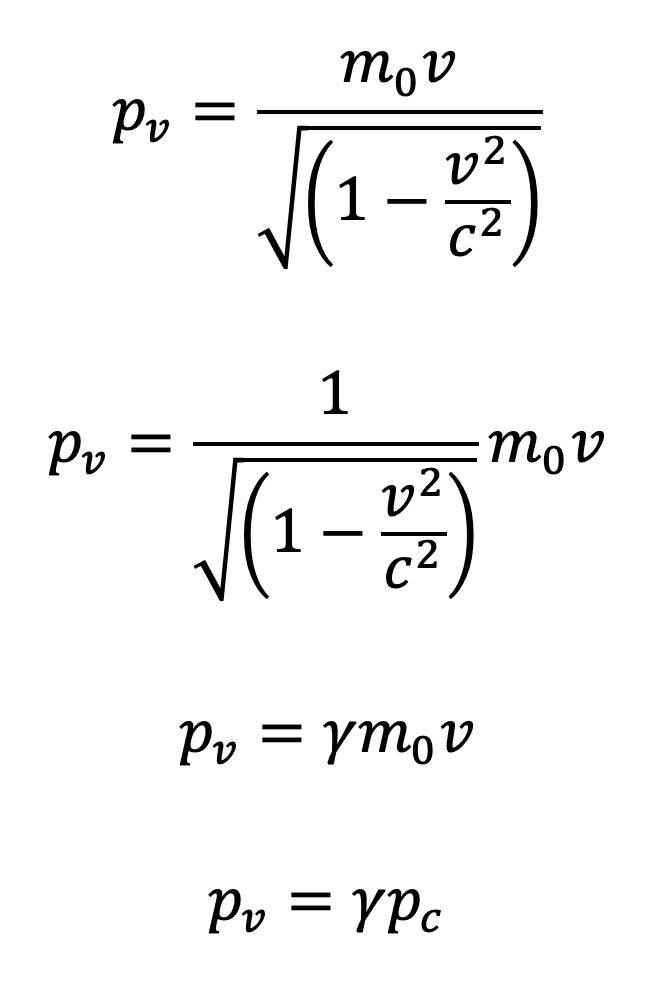Relativistic momentum = relativistic constant ✕ classical momentum

• where γ = is the relativistic constant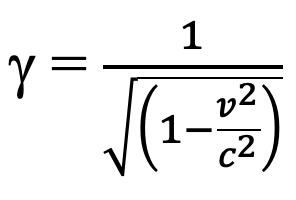When velocity of the observed body is small, relativistic momentum and classical momentum are very similar because is γ close to 1.

Even when a body is travelling at 0.10c, its relativistic momentum would only differ by 0.5%. So, the consequence of special relativity on momentum is not obvious at velocities that we normally observe. Only at a velocity that is 87% of the speed of light, we would observe momentum of a particle twice as its initial.

When velocity approaches the speed of light, relativistic momentum deviates from classical momentum. It increases exponentially.

Kinetic energy at relativistic velocities

Similar to momentum, kinetic energy becomes inconsistent with classical physics when a particle accelerates to very high speeds.Classical theory of kinetic energy states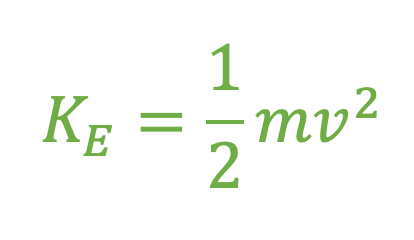Relativistic kinetic energy is calculated differently as Einstein proposes that mass and energy are interchangeable so an increase in mass or energy affects each other.

Thus, the kinetic energy is calculated by considering the work done on a particle from its resting state.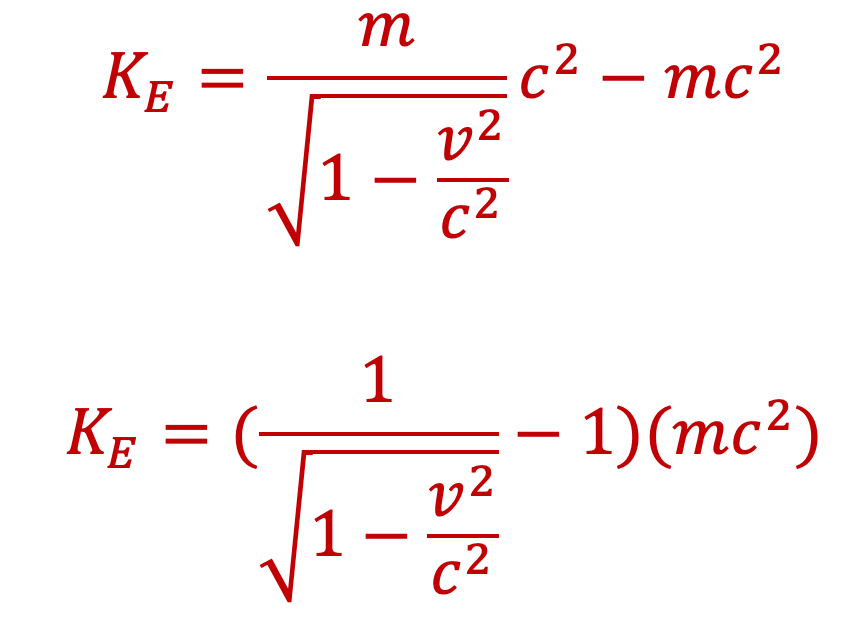As v approaches c, the denominator becomes very small so a particle’s kinetic energy when travelling at a relativistic velocity becomes infinitely large.

This also explains why it is impossible to achieve the speed of light. Therefore, the special theory of relativity again contradicts predictions of classical physics as the speed of light is the absolute maximum speed.

• Energy and mass conservation

In classical physics, the law of energy and mass conservation underpins many common phenomena such as the collision between objects, acceleration of rockets during its launch and all chemical reactions (formation of product from reactants)

In addition to the special theory of relativity, Einstein also proposed that the two quantities (mass and energy) are in fact interchangeable. Mass can be converted into energy and energy can be converted into mass. As a result, each quantity does not need to be conserved in its own form as they can inter-convert.

The mass and energy conversion or equivalence is governed by the equation: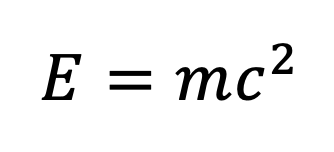• Where E is energy in Joules (J)
• m is the rest mass of a particle or object in kilograms (kg)
• c is the speed of light in ms-2

This form of mass and energy conservation and conversion is commonly observed in nuclear reactions and situations where high-speed particles collide.

• Production of energy by the Sun

Stars, including our Sun, produce energy via nuclear fusion. Nuclear fusion is the process in which atomic nuclei physically combine to form one or more different atomic nucleus and additional sub-atomic particles such as neutrons.

Nuclear fusion obeys energy-mass conservation but requires the application of energy-mass equivalence.

The simplest form of nuclear fusion occurs between hydrogen nuclei to form helium. The atomic mass of one hydrogen atom is roughly 1.008 amu. When four hydrogen atoms combine, the total mass of resultant products should add up to 4.032 amu due to mass conservation.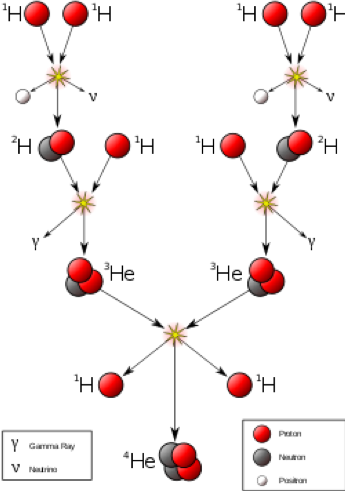However, the resultant helium nucleus weighs less than 4.032 amu which means the lost mass has converted into energy. Using Einstein’s equation and information from the periodic table, the energy produced isThe overall reaction can be summarized by the following equation. Four hydrogen nuclei combine to form one helium nucleus and two positrons. Positrons are essentially positive electrons (you will learn about this in Module 8).It is important to keep in mind that this equation only summarises the process. The formation of helium nucleus through fusion is actually a multi-stage process shown in the diagram on the previous page.

• Binding energy

The process of fusion leads to the formation of new nuclei which require energy to hold nucleons (protons and neutrons) together to prevent decay. This energy is referred to as binding energy.

In a stable isotope e.g. C-12, the total nuclear mass is always lower than the additive masses of its nucleons. A carbon-12 nucleus contains 6 protons and 6 neutrons which add up to a total mass of:However, the actual nuclear mass of C-12 is only 12.00 amu. The mass difference (also known as mass defect) is converted into the binding energy which is required to hold the 12 nucleons together in the nucleus.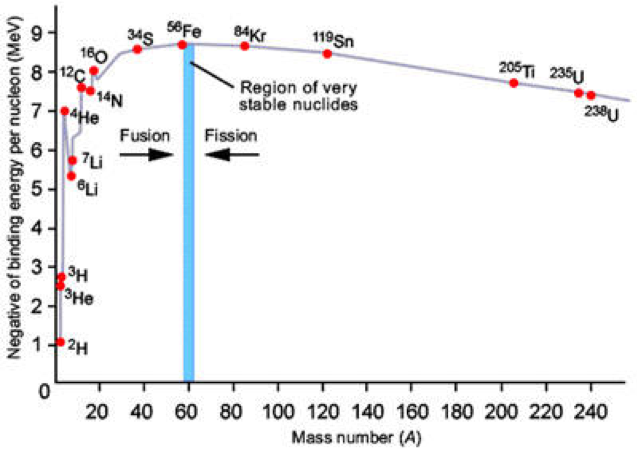• Energy production in particle-antiparticle annihilation

Annihilation is a process in which a particle collides with its anti-particle to produce new particles. An anti-particle is a concept in quantum physics; it is defined as a particle that has quantum numbers which exactly oppose those of the reference particle.

Electron-positron annihilation

Collision between an electron and positron produces two photons with frequencies equivalent to those of gamma radiation. As such the equation is as follows:

The rest energy of an electron and positron are equal because they possess equal mass: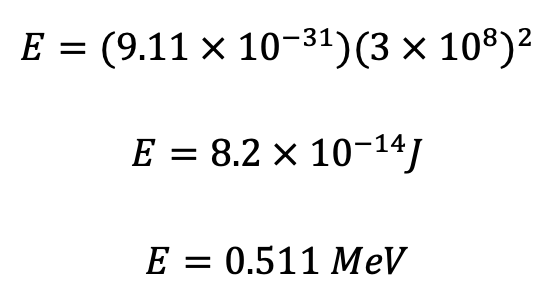The photons produced (gamma rays) each possess the same amount of energy of 0.511 MeV. It is important to note that we can utilise Planck’s quantum theory (E = hf) to calculate the frequency and wavelength associated with a photon of this energy level.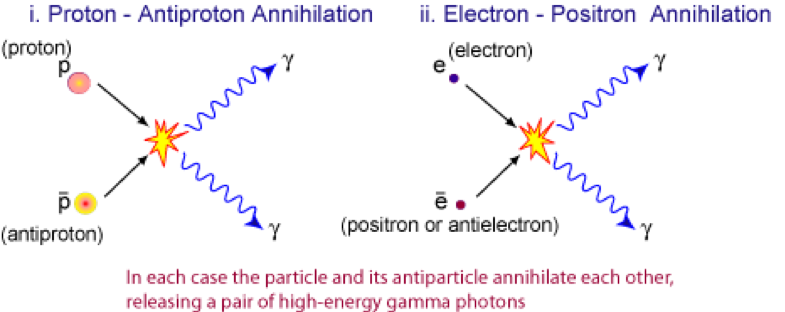Proton-antiproton annihilation

The collision between a proton and antiproton is more complicated than the annihilation between electron and positron. This is because a proton is a baryon consisting of multiple (three to be exact) quarks whereas an electron has a simpler structure.

Due to protons and antiprotons’ more complex structure, the collision involves several interactions and thus, produces more than just photons as energy. The annihilation can also produce electrons, positrons and neutrinos.

• Combustion of fuel

Combustion of fuel is a common application of transforming chemical energy into thermal energy and/or kinetic energy. The difference in mass between products and reactants can be accounted for by considering the energy produced.

Octane is a common fuel you can find at petrol stations. Its combustion reaction is outlined below.The mass of products has been observed to be smaller than the amount of fuel and oxygen gas consumed. However, the mass difference is much harder to determine compared to nuclear reactions as combustion reactions occur on a macroscopic scale.

Practice Question 1

(a) What is the momentum of an electron traveling at a speed 0.985c? The rest mass of the electron is 9.11 × 10-31?

(b) Find the ratio of this momentum to classical momentum of the electron.

Practice Question 2

Is it possible for an external force to be acting on a system and relativistic momentum to be conserved? Explain.

Practice Question 3

Fusion of helium nuclei (nuclear mass = 4.003 amu) can form a carbon nucleus (nuclear mass = 12.000) through the process:

(a) Calculate the mass loss as a result of nuclear fusion. (1 mark)

(b) Hence, determine the energy generated per carbon nucleus formed. (2 marks)

Practice Question 4

Lithium-6 (m = 6.015 amu) can fuse with tritium (hydrogen-3, m = 3.016 amu) to form two nuclei of helium-4 (m = 4.003 amu).

(a) Write an equation to represent this fusion process. (1 mark)

(b) What is the mass defect when lithium-6 is transformed into two nuclei of helium through the reaction above? State your answer in atomic mass units. (1 mark)

(c) Calculate the binding energy of one helium-4 nucleus. (2 marks)

Previous section: Time Dilation and Length Contraction